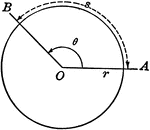### Model of Arc Length, Angle Measure, and Radius

Illustration showing that the arc length can be found by multiplying the angle measure by the radius…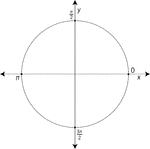### Unit Circle Labeled At Quadrantal Angles

Illustration of a unit circle (circle with a radius of 1) superimposed on the coordinate plane with…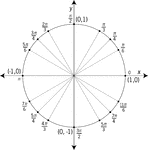### Unit Circle Labeled At Special Angles

Illustration of a unit circle (circle with a radius of 1) superimposed on the coordinate plane with…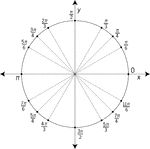### Unit Circle Labeled At Special Angles

Illustration of a unit circle (circle with a radius of 1) superimposed on the coordinate plane with…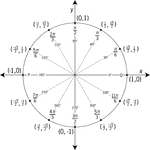### Unit Circle Labeled In 30° Increments With Values

Illustration of a unit circle (circle with a radius of 1) superimposed on the coordinate plane with…### Unit Circle Labeled In 45 ° Increments

Illustration of a unit circle (circle with a radius of 1) superimposed on the coordinate plane with…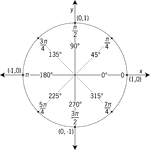### Unit Circle Labeled In 45° Increments With Values

Illustration of a unit circle (circle with a radius of 1) superimposed on the coordinate plane with…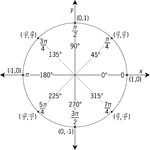### Unit Circle Labeled In 45° Increments With Values

Illustration of a unit circle (circle with a radius of 1) superimposed on the coordinate plane with…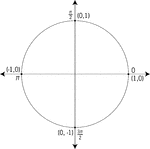### Unit Circle Labeled With Quadrantal Angles And Values

Illustration of a unit circle (circle with a radius of 1) superimposed on the coordinate plane. All…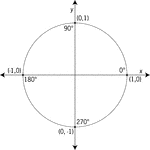### Unit Circle Labeled With Quadrantal Angles And Values

Illustration of a unit circle (circle with a radius of 1) superimposed on the coordinate plane with…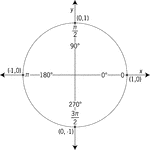### Unit Circle Labeled With Quadrantal Angles And Values

Illustration of a unit circle (circle with a radius of 1) superimposed on the coordinate plane with…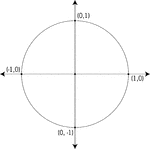### Unit Circle Labeled With Quadrantal Values

Illustration of a unit circle (circle with a radius of 1) superimposed on the coordinate plane. At each…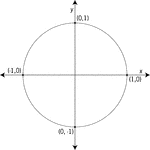### Unit Circle Labeled With Quadrantal Values

Illustration of a unit circle (circle with a radius of 1) superimposed on the coordinate plane with…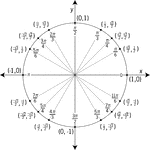### Unit Circle Labeled With Special Angles And Values

Illustration of a unit circle (circle with a radius of 1) superimposed on the coordinate plane with…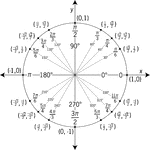### Unit Circle Labeled With Special Angles And Values

Illustration of a unit circle (circle with a radius of 1) superimposed on the coordinate plane with…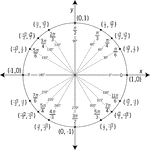### Unit Circle Labeled With Special Angles And Values

Illustration of a unit circle (circle with a radius of 1) superimposed on the coordinate plane with…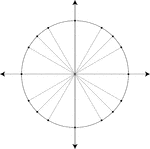### Unit Circle Marked At Special Angles

Illustration of a unit circle (circle with a radius of 1) superimposed on the coordinate plane. All…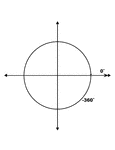### 0° and -360° Coterminal Angles

Illustration showing coterminal angles of 0° and -360°. Coterminal angles are angles drawn in…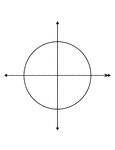### 0° and -360° Coterminal Angles

Illustration showing coterminal angles of 0° and -360°. Coterminal angles are angles drawn in…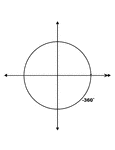### 0° and -360° Coterminal Angles

Illustration showing coterminal angles of 0° and -360°. Coterminal angles are angles drawn in…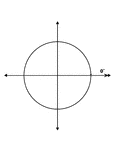### 0° and -360° Coterminal Angles

Illustration showing coterminal angles of 0° and -360°. Coterminal angles are angles drawn in…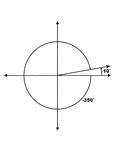### 10° and -350° Coterminal Angles

Illustration showing coterminal angles of 10° and -350°. Coterminal angles are angles drawn…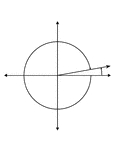### 10° and -350° Coterminal Angles

Illustration showing coterminal angles of 10° and -350°. Coterminal angles are angles drawn…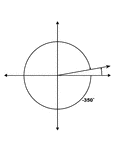### 10° and -350° Coterminal Angles

Illustration showing coterminal angles of 10° and -350°. Coterminal angles are angles drawn…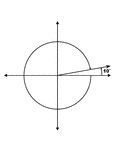### 10° and -350° Coterminal Angles

Illustration showing coterminal angles of 10° and -350°. Coterminal angles are angles drawn…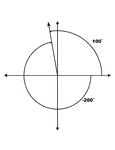### 100° and -260° Coterminal Angles

Illustration showing coterminal angles of 100° and -260°. Coterminal angles are angles drawn…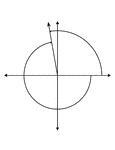### 100° and -260° Coterminal Angles

Illustration showing coterminal angles of 100° and -260°. Coterminal angles are angles drawn…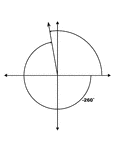### 100° and -260° Coterminal Angles

Illustration showing coterminal angles of 100° and -260°. Coterminal angles are angles drawn…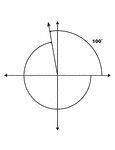### 100° and -260° Coterminal Angles

Illustration showing coterminal angles of 100° and -260°. Coterminal angles are angles drawn…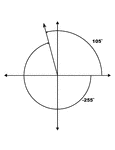### 105° and -255° Coterminal Angles

Illustration showing coterminal angles of 105° and -255°. Coterminal angles are angles drawn…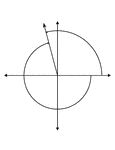### 105° and -255° Coterminal Angles

Illustration showing coterminal angles of 105° and -255°. Coterminal angles are angles drawn…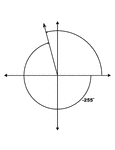### 105° and -255° Coterminal Angles

Illustration showing coterminal angles of 105° and -255°. Coterminal angles are angles drawn…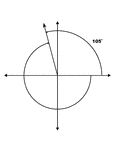### 105° and -255° Coterminal Angles

Illustration showing coterminal angles of 105° and -255°. Coterminal angles are angles drawn…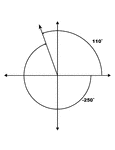### 110° and -250° Coterminal Angles

Illustration showing coterminal angles of 110° and -250°. Coterminal angles are angles drawn…### 110° and -250° Coterminal Angles

Illustration showing coterminal angles of 110° and -250°. Coterminal angles are angles drawn…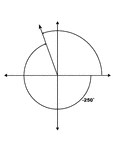### 110° and -250° Coterminal Angles

Illustration showing coterminal angles of 110° and -250°. Coterminal angles are angles drawn…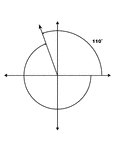### 110° and -250° Coterminal Angles

Illustration showing coterminal angles of 110° and -250°. Coterminal angles are angles drawn…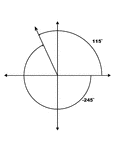### 115° and -245° Coterminal Angles

Illustration showing coterminal angles of 115° and -245°. Coterminal angles are angles drawn…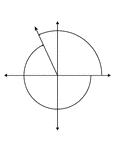### 115° and -245° Coterminal Angles

Illustration showing coterminal angles of 115° and -245°. Coterminal angles are angles drawn…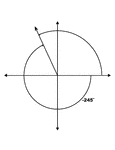### 115° and -245° Coterminal Angles

Illustration showing coterminal angles of 115° and -245°. Coterminal angles are angles drawn…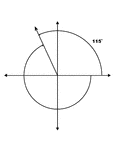### 115° and -245° Coterminal Angles

Illustration showing coterminal angles of 115° and -245°. Coterminal angles are angles drawn…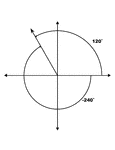### 120° and -240° Coterminal Angles

Illustration showing coterminal angles of 120° and -240°. Coterminal angles are angles drawn…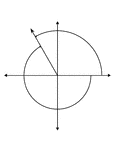### 120° and -240° Coterminal Angles

Illustration showing coterminal angles of 120° and -240°. Coterminal angles are angles drawn…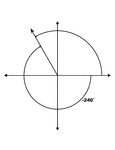### 120° and -240° Coterminal Angles

Illustration showing coterminal angles of 120° and -240°. Coterminal angles are angles drawn…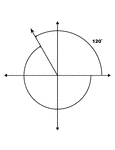### 120° and -240° Coterminal Angles

Illustration showing coterminal angles of 120° and -240°. Coterminal angles are angles drawn…### 125° and -235° Coterminal Angles

Illustration showing coterminal angles of 125° and -235°. Coterminal angles are angles drawn…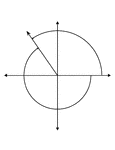### 125° and -235° Coterminal Angles

Illustration showing coterminal angles of 125° and -235°. Coterminal angles are angles drawn…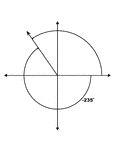### 125° and -235° Coterminal Angles

Illustration showing coterminal angles of 125° and -235°. Coterminal angles are angles drawn…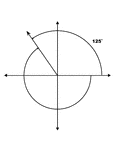### 125° and -235° Coterminal Angles

Illustration showing coterminal angles of 125° and -235°. Coterminal angles are angles drawn…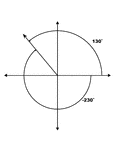### 130° and -230° Coterminal Angles

Illustration showing coterminal angles of 130° and -230°. Coterminal angles are angles drawn…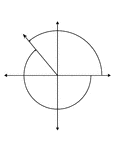### 130° and -230° Coterminal Angles

Illustration showing coterminal angles of 130° and -230°. Coterminal angles are angles drawn…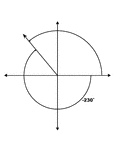### 130° and -230° Coterminal Angles

Illustration showing coterminal angles of 130° and -230°. Coterminal angles are angles drawn…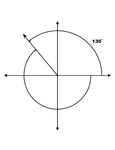### 130° and -230° Coterminal Angles

Illustration showing coterminal angles of 130° and -230°. Coterminal angles are angles drawn…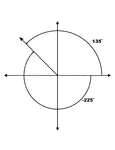### 135° and -225° Coterminal Angles

Illustration showing coterminal angles of 135° and -225°. Coterminal angles are angles drawn…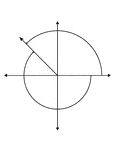### 135° and -225° Coterminal Angles

Illustration showing coterminal angles of 135° and -225°. Coterminal angles are angles drawn…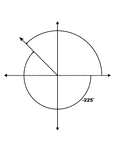### 135° and -225° Coterminal Angles

Illustration showing coterminal angles of 135° and -225°. Coterminal angles are angles drawn…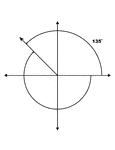### 135° and -225° Coterminal Angles

Illustration showing coterminal angles of 135° and -225°. Coterminal angles are angles drawn…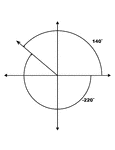### 140° and -220° Coterminal Angles

Illustration showing coterminal angles of 140° and -220°. Coterminal angles are angles drawn…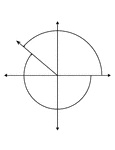### 140° and -220° Coterminal Angles

Illustration showing coterminal angles of 140° and -220°. Coterminal angles are angles drawn…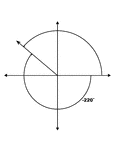### 140° and -220° Coterminal Angles

Illustration showing coterminal angles of 140° and -220°. Coterminal angles are angles drawn…